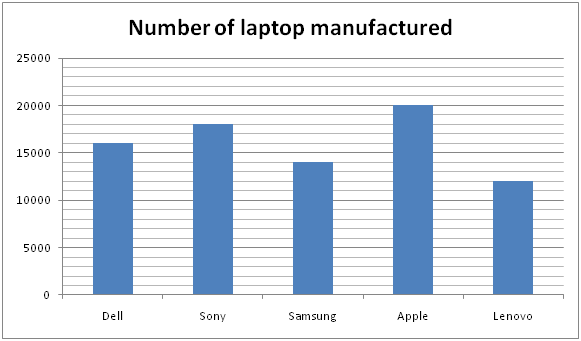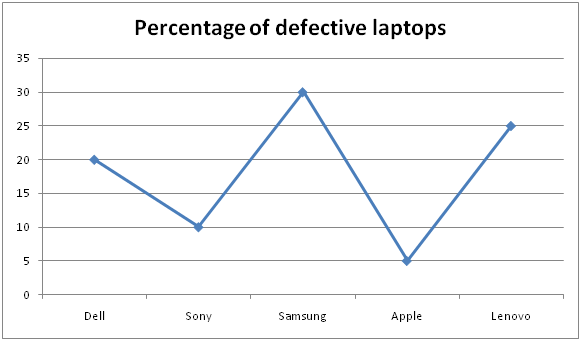# SBI Clerk Prelims 2021 Quantitative Aptitude Questions (Day-25)

Dear Aspirants, Our IBPS Guide team is providing new series of Quantitative Aptitude Questions for SBI Clerk Prelims 2020 so the aspirants can practice it on a daily basis. These questions are framed by our skilled experts after understanding your needs thoroughly. Aspirants can practice these new series questions daily to familiarize with the exact exam pattern and make your preparation effective.

Start Quiz

Bar graph

Directions (01-05): Study the following information carefully and answer the questions given below.

The given bar graph shows the number of manufactured laptops in five different companies.The given line graph shows the percentage of the laptops are defective in five different companies.1) The number of defective Samsung laptops is what percent of the number of non defective Lenovo laptops?

A.42.23%

B.43.34%

C.45.56%

D.46.67%

E.None of these

2) In company Sony, the cost price of a laptop is Rs.40000 and 80% of the non defective laptops are sold. Find the total revenue gets by Sony?

A.Rs.518400000

B.Rs.514800000

C.Rs.524600000

D.Rs.534600000

E.None of these

3) What is the ratio of the number of non defective laptops of company Dell to the number of defective laptops of company Apple?

A.35: 3

B.60: 7

C.64: 5

D.63: 8

E.None of these

4) What is the average number of defective laptops of all the companies together?

A.2520

B.2640

C.2320

D.2780

E.None of these

5) The number of laptops manufactured in Lenovo is what percent of the number of laptops manufactured in Sony?

A.44.45%

B.55.56%

C.66.67%

D.77.78%

E.None of these

Simplification

Directions (06-10): What value should come in place of (?) in the following questions?

6) 125 x 625 ÷ 25 = 5(13 – ?)

A.7

B.8

C.9

D.5

E.6

7) (56 x 0.25)4 ÷ (6860 ÷ 35)4 x (343
8)= (7 x 2)(?) +11

A.3

B.-3

C.4

D.-4

E.5

8) (6 + 66 + 666 + 6666 + 66666) – [22 + {2320 – (256)1/2}1/2] =?

A.64000

B.44000

C.72000

D.84000

E.74000

9) [{(150% of 44) – 11} % of 660] % of 25 = (?) % of 165

A.65

B.45

C.44

D.55

E.75

10) 240% of 20+117÷√81+119+?= 220

A.60

B.50

C.30

D.40

E.None of these

Defective laptops in Samsung = 30/100 * 14000 = 4200

Non defective laptops in Lenovo = 75/100 * 12000 = 9000

Required percentage = 4200/9000 * 100 = 46.67%

Non defective laptops = 90/100 * 18000 = 16200

Sold non defective laptops = 16200 * 80/100 = 12960

Revenue collection = 12960 * 40000 = Rs.518400000

Non defective laptops of Dell = 80/100 * 16000 = 12800

Defective laptops of Apple = 5/100 * 20000 = 1000

Required ratio = 12800: 1000

= 64: 5

Defective laptops of Dell = 20/100 * 16000 = 3200

Defective laptops of Sony = 10/100 * 18000 = 1800

Defective laptops of Samsung = 30/100 * 14000 = 4200

Defective laptops of Apple = 5/100 * 20000 = 1000

Defective laptops of Lenovo = 25/100 * 12000 = 3000

Average = (3200 + 1800 + 4200 + 1000 + 3000)/5 = 2640

Required percentage = 12000/18000 * 100 = 66.67%

53 x 5÷ 5= 5(13 – ?)

7 – 2 = 13 – ?

? = 8

(56 x 0.25)4 ÷ (6860 ÷ 35)4 x (343 x 8)= (7 x 2)(?) +11

(7 x 2)÷ {(7 x 2)2}x {(7 x 2)3}4 = (7 x 2)(x+11)

4 – 8 + 12 = x + 11

x = -11 + 8 = -3

? = 6(1 + 11 + 111 + 1111 + 11111) – (22 + (2320 – 16)1/2)

? = 6(12345) – (22 + 48)

? = 74070 – 70 = 74000

[{66 – 11} % of 660] % of 25 = x % of 165

[55 % of 660] % of 25 = x % of 165

363 % of 25 = x % of 165

? = 9075/165 = 55